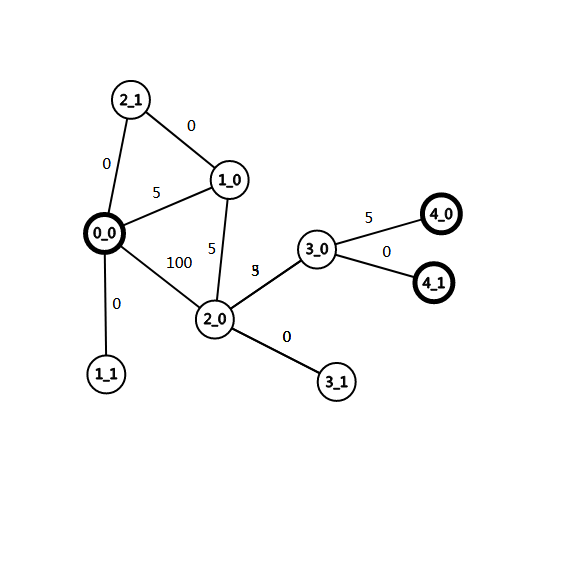2018-10-30 10:42:38$$\text{dis}_{i, j} = \min\{\min\{\text{dis}_{from, j - 1}\}, \min\{\text{dis}_{from,j} + w\}\}$$

struct State { // 优先队列的结点结构体
int v, w, cnt; // cnt 表示已经使用多少次免费通行权限
State() {}
State(int v, int w, int cnt) : v(v), w(w), cnt(cnt) {}
bool operator<(const State &rhs) const { return w > rhs.w; }
};

void dijkstra() {
memset(dis, 0x3f, sizeof dis);
dis[s] = 0;
pq.push(State(s, 0, 0)); // 到起点不需要使用免费通行权，距离为零
while (!pq.empty()) {
const State top = pq.top(); pq.pop();
int u = top.v, nowCnt = top.cnt;
if (done[u][nowCnt]) continue;
done[u][nowCnt] = true;
for (int i = head[u]; i; i = edge[i].next) {
int v = edge[i].v, w = edge[i].w;
if (nowCnt < k && dis[v][nowCnt + 1] > dis[u][nowCnt]) { // 可以免费通行
dis[v][nowCnt + 1] = dis[u][nowCnt];
pq.push(State(v, dis[v][nowCnt + 1], nowCnt + 1));
}
if (dis[v][nowCnt] > dis[u][nowCnt] + w) { // 不可以免费通行
dis[v][nowCnt] = dis[u][nowCnt] + w;
pq.push(State(v, dis[v][nowCnt], nowCnt));
}
}
}
}

int main() {
// 笔者习惯从 1 到 n 编号，而这道题是从 0 到 n - 1，所以要处理一下
}